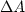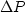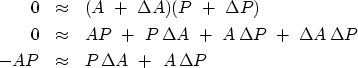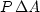Next: Spectral preference and training Up: MISSING DATA AND UNKNOWN Previous: Objections to interpolation error

## Packing both missing data and filter into a CG vector

Now let us examine the theory and coding behind the above examples. Define a roughening filter A(Z) and a data signal P(Z) at some stage of interpolation. The regression iswhere the filter A(Z) has at least one coefficient constrained to be nonzero and the data contains both known and missing values. Think of perturbationsand.We neglect the nonlinear termas follows:(1) (2) (3)
To make a program such as miss1(), we need to pack both unknowns into a single vector x() =before calling the conjugate-gradient program. Likewise, the resulting filter and data coming out must be unpacked. Also, the gradient now has two contributions, one fromand one from,and these must be combined. The program missif(), which makes Figures 8 through 11, effectively combines miss1()and iner(). A new aspect is that, to avoid accumulation of errors from the neglect of the nonlinear product,the residual is recalculated inside the iteration loop instead of only once at the beginning.

# MISSIF - find MISSing Input data and Filter on 1-axis by min power out.
#
subroutine missif( na, lag, aa, np, pp, known, niter)
integer iter,      na, lag,     np,            niter, nx, ax, px, ip, nr
real    pp(np)          # input: known data with zeros for missing values.
# output: known plus missing data.
real    known(np)       # known(ip) vanishes where p(ip) is a missing value.
real    aa(na)          # input and output: roughening filter
temporary real  x(np+na), g(np+na), s(np+na)
temporary real  rr(np+na-1), gg(np+na-1), ss(np+na-1)
nr= np+na-1;    nx= np+na;      px=1;   ax=1+np;
call copy( np,  pp, x(px))
call copy( na,  aa, x(ax))
if( aa(lag) == 0. )     call erexit('missif: a(lag)== 0.')
do iter= 0, niter {
call contran( 0, 0, na,aa, np, pp,    rr)
call scaleit  (         -1., nr,        rr)
call contran( 1, 0, na,aa, np, g(px), rr)
call contran( 1, 0, np,pp, na, g(ax), rr)
do ip= 1, np
if( known(ip) != 0)
g( ip) = 0.
g( lag+np) = 0.
call contran( 0, 0, na,aa, np, g(px), gg)
call contran( 0, 1, np,pp, na, g(ax), gg)
call cgstep( iter, nx, x, g, s, nr, rr, gg, ss)
call copy( np, x(px), pp)
call copy( na, x(ax), aa)
}
return; end


There is a danger that missif() might converge very slowly or fail if aa() and pp() are much out of scale with each other, so be sure you input them with about the same scale. I really should revise the code, perhaps to scale the 1'' in the filter to the data, perhaps to equal the square root of the sum of the data values.Next: Spectral preference and training Up: MISSING DATA AND UNKNOWN Previous: Objections to interpolation error
Stanford Exploration Project
10/21/1998